• 点云法向量估计
千次阅读
2021-07-25 12:10:46

## 1 法向量估计函数 pcnormals

pcnormals 函数用于点云法向量估计，MATLAB提供了两种重载方式

normals = pcnormals(ptCloud)


返回一个矩阵，该矩阵的元素对应为输入点云ptCloud中的每个点的法线。该函数使用六个相邻点拟合局部平面来确定每个法向量。

normals = pcnormals(ptCloud,k)


指定用于局部平面拟合的点数k，若不指定，则默认 k = 6

更多相关内容
• opencv 平面法向量_任意两平面求夹角 import math#引入math模块 计算角度用 class point(object):#定义空间点类 """docstring for point""" def __init__(self,x,y,z,name): self.x = x self.y = y self...

目录

单通道深度图像，转三通道法向图像

opencv 平面法向量_任意两平面求夹角

点云3种法向量估计方法及可视化

###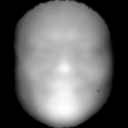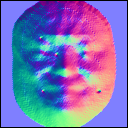import cv2
import numpy as np
import mxnet as mx
def depth2normal(depth):
w,h=depth.shape
dx=-(depth[2:h,1:h-1]-depth[0:h-2,1:h-1])*0.5
dy=-(depth[1:h-1,2:h]-depth[1:h-1,0:h-2])*0.5
dz=mx.nd.ones((w-2,h-2))
dl = mx.nd.sqrt(mx.nd.elemwise_mul(dx, dx) + mx.nd.elemwise_mul(dy, dy) + mx.nd.elemwise_mul(dz, dz))
dx = mx.nd.elemwise_div(dx, dl) * 0.5 + 0.5
dy = mx.nd.elemwise_div(dy, dl) * 0.5 + 0.5
dz = mx.nd.elemwise_div(dz, dl) * 0.5 + 0.5
return np.concatenate([dy.asnumpy()[np.newaxis,:,:],dx.asnumpy()[np.newaxis,:,:],dz.asnumpy()[np.newaxis,:,:]],axis=0)
if __name__ == '__main__':
normal=np.array(depth2normal(mx.nd.array(depth))*255)
normal = cv2.cvtColor(np.transpose(normal, [1, 2, 0]), cv2.COLOR_BGR2RGB)
cv2.imwrite("normal.png",normal.astype(np.uint8))

原文链接：https://blog.csdn.net/muyouhang/article/details/103649888

### opencv 平面法向量_任意两平面求夹角

import math#引入math模块 计算角度用
class point(object):#定义空间点类
"""docstring for point"""
def __init__(self,x,y,z,name):
self.x = x
self.y = y
self.z = z
self.name = name
class plane(object):#定义平面类
"""docstring for plane"""
def __init__(self, A,B,C,name):
self.points=[A,B,C]#一个平面三个点
self.points_name=[A.name,B.name,C.name]#点的名字
self.name = name#平面的名字
self.n=[]#平面的法向量
def isplane(self):#判断这三个点是否能构成平面
coors=[[],[],[]]#三个点的xyz坐标分别放在同一个列表用来比较
for _point in self.points:#对于每个点
coors.append(_point.x)
coors.append(_point.y)
coors.append(_point.z)
for coor in coors:
if coor==coor==coor:#如果三个点的x或y或z坐标相等 则不能构成平面
return print('Points:',*self.points_name,'cannot form a plane')
def normal(self):#获得该平面的法向量
self.isplane()#获得该平面的法向量前提是能构成平面
A,B,C=self.points#对应三个点
AB=[B.x-A.x,B.y-A.y,B.z-A.z]#向量AB
AC=[C.x-A.x,C.y-A.y,C.z-A.z]#向量AC
B1,B2,B3=AB#向量AB的xyz坐标
C1,C2,C3=AC#向量AC的xyz坐标
self.n=[B2*C3-C2*B3,B3*C1-C3*B1,B1*C2-C1*B2]#已知该平面的两个向量,求该平面的法向量的叉乘公式
def angle(self,P2):#两个平面的夹角
x1,y1,z1=self.n#该平面的法向量的xyz坐标
x2,y2,z2=P2.n#另一个平面的法向量的xyz坐标
cosθ=((x1*x2)+(y1*y2)+(z1*z2))/(((x1**2+y1**2+z1**2)**0.5)*((x2**2+y2**2+z2**2)**0.5))#平面向量的二面角公式
degree=math.degrees(math.acos(cosθ))
if degree>90:#二面角∈[0°,180°] 但两个平面的夹角∈[0°,90°]
degree=180-degree
return print('平面',self.name,P2.name,'的夹角为'+str(round(degree,5))+'°')
#round(数值,四舍五入位数) math.degrees(弧度)将弧度转换为角度 math.acos(数值)返回该数值的反余弦弧度值
#测试
print('-'*25)
A=point(0,0,1,'A')#六个点
B=point(1,0,1,'B')
C=point(1,1,0,'C')
P1=plane(A,B,C,'P1')#p1平面
D=point(0,1,1,'D')
E=point(1,1,1,'E')
F=point(0.5,0,0,'F')
P2=plane(D,E,F,'P2')#p2平面
P1.normal()#求平面p1 p2的法向量
P2.normal()
P1.angle(P2)#求平面p1 p2的夹角
print('-'*25)
G=point(2,0,0,'G')#六个点
H=point(0,0,0,'H')
I=point(0,3,3**0.5,'I')
P3=plane(G,H,I,'P3')#p3平面
J=point(2/3,4/3,0,'J')
K=point(0,0,0,'K')
L=point(0,3,3**0.5,'L')
P4=plane(J,K,L,'P4')#p4平面
P3.normal()#分别求平面p3 p4的法向量
P4.normal()
P3.angle(P4)#求平面p3 p4的夹角
print('-'*25)
M=point(0,1,0,'M')#六个点
N=point(0,0,0,'N')
O=point(1,1,1,'O')
P5=plane(M,N,O,'P5')#p1平面
Q=point(0,0,2,'Q')
R=point(0,0,0,'R')
S=point(1,1,1,'S')
P6=plane(Q,R,S,'P6')#p2平面
P5.normal()#求平面p1 p2的法向量
P6.normal()
P5.angle(P6)#求平面p1 p2的夹角
print('-'*25)
T=point(12.6,-1,63,'T')#六个点
U=point(0,7,8,'U')
V=point(11,9,83.2,'V')
P7=plane(T,U,V,'P7')#p1平面
W=point(45,2,13,'W')
X=point(9,10,-56,'X')
Y=point(0.5,-7,1,'Y')
P8=plane(W,X,Y,'P8')#p2平面
P7.normal()#求平面p1 p2的法向量
P8.normal()
P7.angle(P8)#求平面p1 p2的夹角



原文链接：https://blog.csdn.net/weixin_36313588/article/details/112189888

# 1）点云读取可视化

原始点云：# 2）下采样可视化

下采样：# 3）法向量三种估计方式（K近邻估计，半径近邻估计，混合搜索估计）

K近邻估计法向量并可视化：混合搜索近邻估计可视化# 4）点云每个点对应的法向量点存储及可视化

法向量对应的点可视化：# 5）法向量点和原始点云同时可视化

原始点云灰色，法向量点绿色# 6） 源码

# @Description: <Open3D估计法向量，可视化，存储为文件>

import open3d as o3d
import os

path = os.path.abspath(os.path.join(os.getcwd(), "../"))
path = path + "/pcds/bunny.pcd"
normalPath = path.replace(".pcd", "_normal.pcd")
print(path)
print(normalPath)

print("Load a pcd point cloud, print it, and render it")
pcd.paint_uniform_color([0.5, 0.5, 0.5])  # 把所有点渲染为灰色（灰兔子）
print(pcd)  # 输出点云点的个数
# print(o3d.np.asarray(pcd.points))  # 输出点的三维坐标
o3d.visualization.draw_geometries([pcd], "Open3D origin points", width=800, height=600, left=50, top=50,
point_show_normal=False, mesh_show_wireframe=False,
mesh_show_back_face=False)

print("Downsample the point cloud with a voxel of 0.002")
downpcd = pcd.voxel_down_sample(voxel_size=0.002)  # 下采样滤波，体素边长为0.002m
print(downpcd)
o3d.visualization.draw_geometries([downpcd], "Open3D downsample points", width=800, height=600, left=50, top=50,
point_show_normal=False, mesh_show_wireframe=False,
mesh_show_back_face=False)

print("Recompute the normal of the downsampled point cloud")
# 混合搜索  KNN搜索  半径搜索
# downpcd.estimate_normals(
downpcd.estimate_normals(
search_param=o3d.geometry.KDTreeSearchParamKNN(knn=20))  # 计算法线，只考虑邻域内的20个点
# downpcd.estimate_normals(

o3d.visualization.draw_geometries([downpcd], "Open3D normal estimation", width=800, height=600, left=50, top=50,
point_show_normal=True, mesh_show_wireframe=False,
mesh_show_back_face=False)  # 可视化法线
print("Print a normal vector of the 0th point")
print(downpcd.normals)  # 输出0点的法向量值

print("Print the normal vectors of the first 10 points")
print(o3d.np.asarray(downpcd.normals)[:10, :])  # 输出前10个点的法向量

# std::vector<Eigen::Vector3d> with 381 elements. 转换为nparry 以打印访问
# normals = o3d.np.asarray(downpcd.normals)
# print(normals)

# 可视化法向量的点，并存储法向量点到文件
normal_point = o3d.utility.Vector3dVector(downpcd.normals)
normals = o3d.geometry.PointCloud()
normals.points = normal_point
normals.paint_uniform_color((0, 1, 0))  # 点云法向量的点都以绿色显示
o3d.visualization.draw_geometries([pcd, normals], "Open3D noramls points", width=800, height=600, left=50, top=50,
point_show_normal=False, mesh_show_wireframe=False,
mesh_show_back_face=False)
o3d.io.write_point_cloud(normalPath, normals)
展开全文• 法向量三种估计方式（K近邻估计，半径近邻估计，混合搜索估计） 差别只在于搜索近邻点的方法略微有差异，实际上差别不大； # @Description: <Open3D估计法向量，可视化，存储为文件> import open3d as ...

法向量三种估计方式（K近邻估计，半径近邻估计，混合搜索估计）

差别只在于搜索近邻点的方法略微有差异，实际上差别不大；

# @Description: <Open3D估计法向量，可视化，存储为文件>

import open3d as o3d
import os

path = os.path.abspath(os.path.join(os.getcwd(), "../"))
path = path + "/pcds/bunny.pcd"
normalPath = path.replace(".pcd", "_normal.pcd")
print(path)
print(normalPath)

print("Load a pcd point cloud, print it, and render it")
pcd.paint_uniform_color([0.5, 0.5, 0.5])  # 把所有点渲染为灰色（灰兔子）
print(pcd)  # 输出点云点的个数
# print(o3d.np.asarray(pcd.points))  # 输出点的三维坐标
o3d.visualization.draw_geometries([pcd], "Open3D origin points", width=800, height=600, left=50, top=50,
point_show_normal=False, mesh_show_wireframe=False,
mesh_show_back_face=False)

print("Downsample the point cloud with a voxel of 0.002")
downpcd = pcd.voxel_down_sample(voxel_size=0.002)  # 下采样滤波，体素边长为0.002m
print(downpcd)
o3d.visualization.draw_geometries([downpcd], "Open3D downsample points", width=800, height=600, left=50, top=50,
point_show_normal=False, mesh_show_wireframe=False,
mesh_show_back_face=False)

print("Recompute the normal of the downsampled point cloud")
# 混合搜索  KNN搜索  半径搜索
# downpcd.estimate_normals(
downpcd.estimate_normals(
search_param=o3d.geometry.KDTreeSearchParamKNN(knn=20))  # 计算法线，只考虑邻域内的20个点
# downpcd.estimate_normals(

o3d.visualization.draw_geometries([downpcd], "Open3D normal estimation", width=800, height=600, left=50, top=50,
point_show_normal=True, mesh_show_wireframe=False,
mesh_show_back_face=False)  # 可视化法线
print("Print a normal vector of the 0th point")
print(downpcd.normals)  # 输出0点的法向量值

print("Print the normal vectors of the first 10 points")
print(o3d.np.asarray(downpcd.normals)[:10, :])  # 输出前10个点的法向量

# std::vector<Eigen::Vector3d> with 381 elements. 转换为nparry 以打印访问
# normals = o3d.np.asarray(downpcd.normals)
# print(normals)

# 可视化法向量的点，并存储法向量点到文件
normal_point = o3d.utility.Vector3dVector(downpcd.normals)
normals = o3d.geometry.PointCloud()
normals.points = normal_point
normals.paint_uniform_color((0, 1, 0))  # 点云法向量的点都以绿色显示
o3d.visualization.draw_geometries([pcd, normals], "Open3D noramls points", width=800, height=600, left=50, top=50,
point_show_normal=False, mesh_show_wireframe=False,
mesh_show_back_face=False)
o3d.io.write_point_cloud(normalPath, normals)

展开全文python c++
• ## 3D【24】PCA点云法向量估计

万次阅读 多人点赞 2018-06-05 19:50:09
基于PCA的点云法向量估计，其实是从最小二乘法推导出来的。假设我们要估计某一点的法向量，我们需要通过利用该点的近邻点估计出一个平面，然后我们就能计算出该点的法向量。或者可以这么说，通过最小化一个目标函数...

点云法向量的估计在很多场景都会用到，比如ICP配准，以及曲面重建。

基于PCA的点云法向量估计，其实是从最小二乘法推导出来的。假设我们要估计某一点的法向量，我们需要通过利用该点的近邻点估计出一个平面，然后我们就能计算出该点的法向量。或者可以这么说，通过最小化一个目标函数（要求的参数为法向量），使得该点与其每个近邻点所构成的向量与法向量的点乘为0，也就是垂直：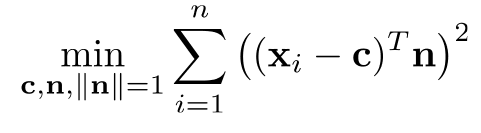正常情况下，我们可以将点c看成是某一领域中所有点的中心点：

m=1ni=1nXi m = 1 n ∑ i = 1 n X i

同时：

yi=Xim y i = X i − m

这样，优化目标函数变为：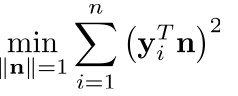我们进一步对目标函数进行推导：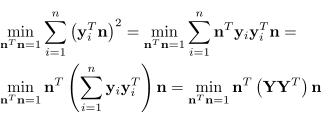上面的优化目标函数等于：

f(n)=nTSnS=(YYT)min(f(n))s.t.nTn=1 f ( n ) = n T S n 其 中 ， S = ( Y Y T ) m i n ( f ( n ) ) s . t . n T n = 1

其中， YYT Y Y T $YY^T$ 是一个3×3的协方差矩阵，x，y，z坐标的协方差矩阵。
对于这种有约束的优化目标函数，我们可以用拉格朗日算法进行求解：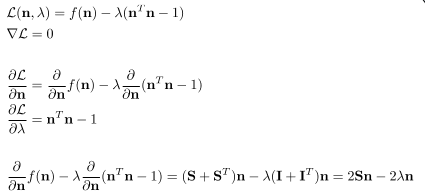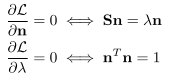Sn=λn S n = λ n $Sn=\lambda n$?有没有很眼熟，其实就是线性代数里面的特征值和特征向量的相关公式。也即是， λ λ $\lambda$ 是矩阵S的特征值，法向量是对应的特征向量。

所以法向量n的求解就是要对S进行向量分解。然后取特征值最小的特征向量作为求解的法向量。这个过程也就是PCA的一个标准求解过程，将每个邻域点与该邻域的中心点相减，得到一个n×3的矩阵。接着，用SVD对该矩阵进行分解，得到：

Y=UΣV Y = U Σ V ∗

U中最后一列就是要求解的法向量n，也就是特征值最小的特征向量。

为什么是特征值最小的？我个人理解是：PCA的协方差矩阵的特征值越大，其特征向量就越能够描述数据的特征。越小就越不能区分样本之间的不同，也就是表征了数据中的共性。在我们的优化目标函数中，就是要为所有的邻域点寻找一个平面，使得所有的邻域点都在这个平面上，或者说所有点与该平面的距离最小，而不是而不是最大。而这个平面就是能够描述这些点的共性，即他们的法向量都一样。

也可以用另外一个求法，直接对协方差矩阵S，求解特征值和特征向量，然后取最小的特征值对应的特征向量。另外，也不一定用中心点m作为c，可以用点云中要求解法向量的顶点作为顶点c。

在求出法向量后还需要判断法向量的方向，一般来说法向量方向的矫正没有严格的数学公式可以使用。比较简单的方法是通过视点方向进行判断。比如我的视点方向是（0,0,1），那么求出来的法向量跟（0,0,1）进行点乘，如果小于0，就将法向量取反。更复杂的方法是使用最小生成树对法向量进行方向矫正。

展开全文• 1、点云法向量估计的主要思路是对K-近邻的N个点进行平面拟合（平面过N点重心），平面法向量即为所求； 2、最小二乘拟合可以转换为求协方差矩阵最小特征值对应的特征向量（SVD分解）；此种解法对数据噪声有很强的...
• 该博客主要进行： （1）点云读取可视化 （2）下采样可视化 （3）法向量三种估计方式（K近邻估计，半径近邻估计，混合搜索估计） （4）点云法向量可视化 （5）点云每个点对应的法向量点存储 （6）点云法向量点可视化python Open3D
• 基于PCL的三维点云模型表面法向量估计的具体实现
• PCL教程指南-Estimating Surface Normals in a PointCloud(估计点云法向量) 官方原文档 点云法向指每个点的法向量，它是基于各点所在邻域范围内估计而出，常用方法如最小二乘估计邻域平面进而求出法向量，PCA求解...
• 基于局部平面拟合求点云法向量，是我看到过的比较容易懂的，算法推导也写得很清楚
• ## 点云法向量

千次阅读 2022-04-23 11:02:13open3d python
• 估计每个顶点的法向量，单独从一点来看是无法做到的，一般是找到邻域，以邻域拟合平面近似估计顶点法向量。 从计算的角度来看，计算法向量首先连接邻域到顶点，得到一系列向量ViViVi，然后假设一个法向量n⃗\vec{n}n...计算机视觉
• 核心代码 void pcaNormal(const PointCloud<PointXYZ>::Ptr& cloud, const int& k_neighbors, PointCloud<Normal>::Ptr& normals) //cloud为所求取的点云，k_neighbors为输入的Kd树的k值，normals为点云法向量 { /...c++ 计算机视觉 自动驾驶
• Output:主成分向量 ，principle vectors ，仅仅是一个向量，代表一个方向。主成分的个数k<=原始空间维度数d Q&A 什么是最主要的成分？ 点的投影后分布的方差最大的方向。(在该方向上的点云分布的最分散) ...PCA
• [PCL] 点云法向量计算NormalEstimation 转载来源 从GitHub的代码版本库下载源代码https://github.com/PointCloudLibrary/pcl，用CMake生成VS项目，查看PCL的源码位于pcl_features项目下 1.Feature类： template <...
• 但通过一组实际获取的真实表面点云数据来进行相应的法向量估计，通常有两种方案： 使用表面重建技术，针对获取的点运数据集，从网格化后的重建表面上估计法向量。 直接从点云数据中估计法向量。 直接从点云数据中...
• import pcl import pcl.pcl_visualization def main(): cloud = pcl.load('filename.pcd') # load the pcd file ne = cloud.make_NormalEstimation() # instance the NormalEstimation ne.set_KSearch(20) # ...python
• 该论文详细论述了三维点云法向量估计算法及研究现状，对从事三维重建方面工作的人员有很好的帮助。
•c++
• 转自：点云的曲面法向量估计表面法线是几何体表面的重要属性，在很多领域都有大量应用，例如：在进行光照渲染时产生符合可视习惯的效果时需要表面法线信息才能正常进行，对于一个已知的几何体表面，根据垂直于点表面...
• 用主成分分析法估计出点云中每点的法向量函数，实现平台为matlab
• 1. 估算PointCloud的表面法线（Surface Normals） 2. 打印点云法向量结果； 3. 存储点云法向量结果；python
• 本文介绍了PCL法线估计并可视化的方法，同时给出了OpenMP加速法线估计算法
• ## PCL估计点云的表面法向量

万次阅读 多人点赞 2019-06-26 10:37:18
PCL估计点云的表面法向量估计点云表面法向量的方法理论基础法线确定方法法线方向确定选择合适的邻域尺度PCL估计表面法线代码实现用OpenMP加速法线估计参考资料 估计点云表面法向量的方法 根据点云中点及其邻域...
• 原理 PCA原理 ...3D【24】PCA点云法向量估计 利用PCA计算点云的法线 3D点云法向量估计(最小二乘拟合平面) 为什么用PCA做点云法线估计？ 利用PCA求点云的法向量 pca_demo.m clc clear cl...matlab pca
• 最近报了个点云学习的网课，前段时间因为各种问题，落下了很多课程，今天开始要好好搞起来了~~ python真的有点难，之前很少用，也借着这次课程好好学习一下python 虽然PCL一些现成的库里面封装了PCA，但是自己写......